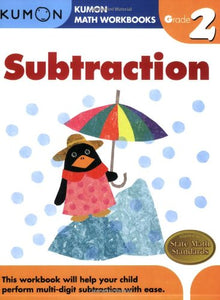Kumon

# Kumon Math Workbooks Grade 2: Subtraction

Regular price \$13.80 \$0.00 Unit price per
Shipping calculated at checkout.

This workbook helps your child subtract three-digit numbers with confidence. Children will learn to regroup (borrow) when subtracting, which equips them with the tools to subtract three-digit numbers. Vertical subtraction is included along with patterning and sequencing activities, so children gain a fundamental understanding of subtraction.
Topics Covered in this Book:

Horizontal subtraction review
Vertical form subtraction
Regrouping (borrowing)
Three-digit minus two-digit subtraction
Early three- & four-digit subtraction
Addition & subtraction of three two-digit numbers
Patterns & sequencing